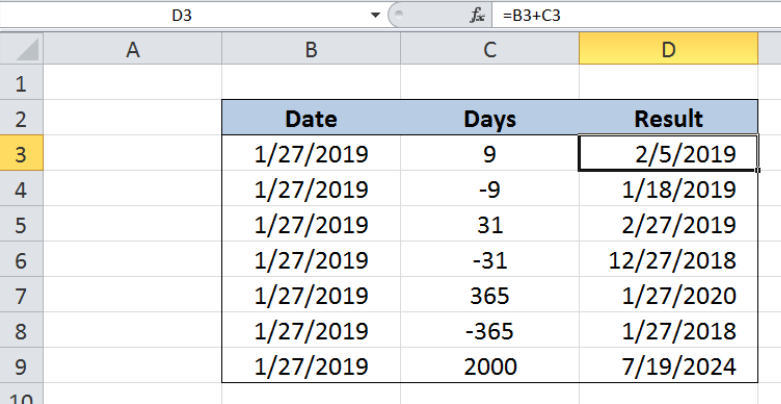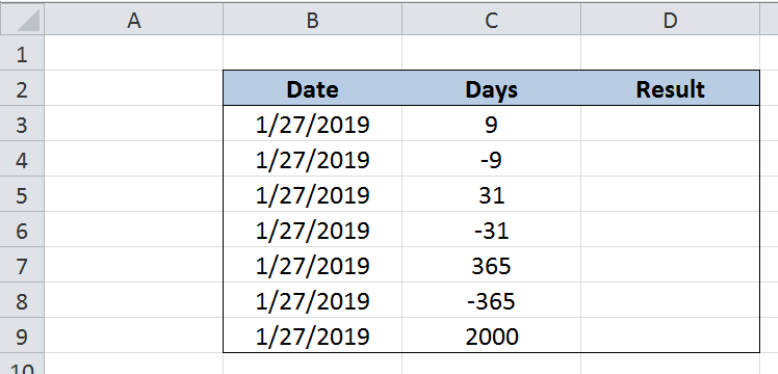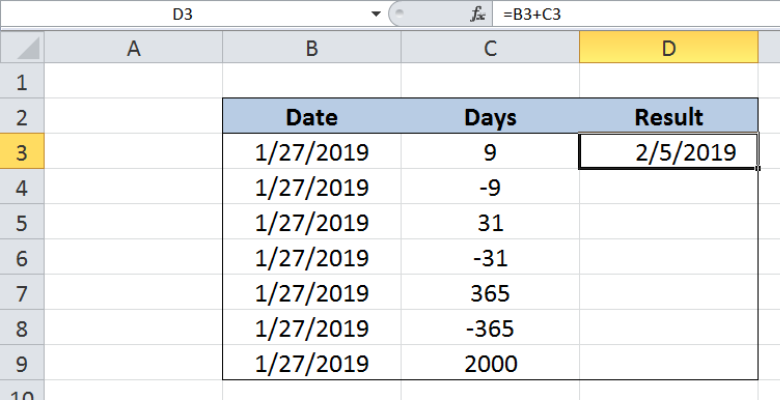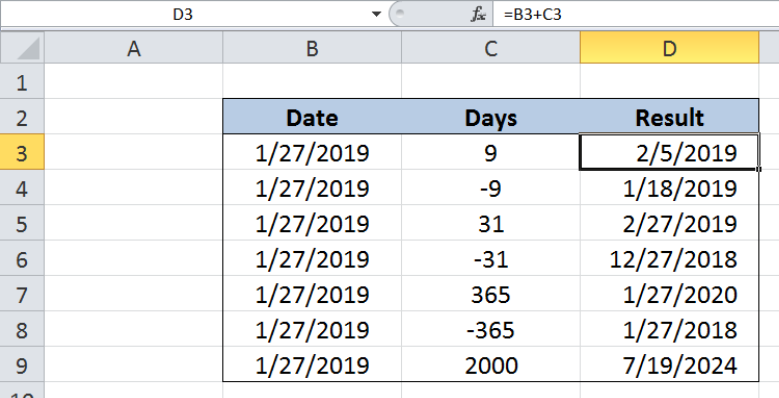Get instant live expert help with Excel or Google Sheets“My Excelchat expert helped me in less than 20 minutes, saving me what would have been 5 hours of work!”

#### Post your problem and you’ll get Expert help in seconds.

Your message must be at least 40 characters
Our professional Expert are available now. Your privacy is guaranteed.

Excel uniquely stores dates as serial numbers, making it easy to perform calculations with dates.  Adding or subtracting days can be done by using one of the basic arithmetic operations, addition. This tutorial will assist all levels of Excel users in adding days to date.Figure 1.  Final result: Add days to date

## Setting up our Data

Our table contains three columns: Date (column B), Days (column C) and Result (column D).  We want to add or subtract the number of days in column C from the start date in column B by using a simple mathematical operation, addition.  The results will be recorded in column D.Figure 2.  Sample data to add days to date

Excel has a unique way of storing dates as serial numbers where the value “1” corresponds to the date “January 1, 1900”.  We want to add days to a start date by simply using addition. We can do this by following these steps:

Step 1.  Select cell D3

Step 2.  Enter the formula:` =B3+C3  `

Step 3.  Press ENTER

Step 4:  Copy the formula in cell D3 to cells D4:D9 by clicking the “+” icon at the bottom-right corner of cell D3 and dragging it downFigure 3.  Entering the formula to add days to date

As long as a date is valid, we can directly add or subtract days to obtain results.  A positive value for days refers to a future date while a negative value pertains to a past date.  Excel is able to perform calculations involving dates because each date is stored as a serial number.

For our first example, 1/27/2019 has the serial number 43492.  We add 9 days and the result is the date “2/5/2019”, whose serial number is 43501.  Our formula looks like this:

`43492 + 9  = 43501`

When formatted as a date, the serial number 43501 is displayed as the date “2/5/2019”.

For the next example, we subtract 9 days to the start date.  As a result, the date in D4 is “1/18/2019”.

See below filled-up table that shows the results when the number of days in column C is added to the start date in column B, by using simple addition.Figure 4.  Output: Add days to date

Most of the time, the problem you will need to solve will be more complex than a simple application of a formula or function. If you want to save hours of research and frustration, try our live Excelchat service! Our Excel Experts are available 24/7 to answer any Excel question you may have. We guarantee a connection within 30 seconds and a customized solution within 20 minutes.

### Did this post not answer your question? Get a solution from connecting with the expert.Another blog reader asked this question today on Excelchat:## Subscribe to Excelchat.coAnother blog reader asked this question today on Excelchat: